# Low Loss "A-Core"

### A NEW GENERATION IN TRANSFORMER DESIGN

Tape wound cut cores throughout the core manufacturing industries are currently being produced with a straight cut along the horizontal plane as core shown in Figure 1 below.

The core is cut in this manner so that a coil designed with specific winding turns and dimensions can be placed onto the leg of the core as part of an assembly unit for a transformer (see Figure 2).Cut cores require large magnetizing current that is necessary to magnetize the core and also to jump across the air gap between the cut segments of area D x E This current is often referred to as the Excitation Current (Iex ) and is defined as:

Equation 1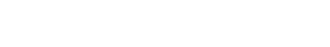Equation 2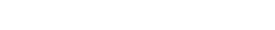wt = Weight of the core in pounds VA/# = Volt Ampere / pound of core material V = Voltage applied to the winding N B = Flux Density eg = Effective air gap between the cut segments SF = Stacking Factor N = Number of Turns of primary winding Imat = Current to magnetize material Igap = Current to drive flux over air gap

Equations (I) and (2) show that the excitation current Iex is dependent on the material quality (VA/#), and the effective air gap eg between the cut segments.

For many applications such as Power Transformers, AC Inductors, and Current Transformers etc., it is necessary to minimize the current Iex of the core. Therefore, besides selecting the right material for the application, the air gap of the cores has to be kept as small as possible to achieve a maximum efficiency rating. Usually core manufacturers lap the mating core surfaces to reduce the air gap, but lapping has its limitations, and will greatly increase the cost of the core.

Example: A core weighing 0.5 to 2.0 lbs normally has air gap of .001". By precision lapping the two segments of the core, the typical gap achievable would be .0005". This is only a small difference in the gap, but the added cost for the lapping is significant.

### The Cut-Angle Core or A-Core (patent pending)

Figures 3 and 4- will minimize the effective air gap eg in a low cost manner by increasing the matching surface area of the two segments and thus reducing the reluctance. The matching surface area of a straight cut core is defined as: A = Dx E. The matching surface area of a Cut angle (Figures 3 and 4) is defined as: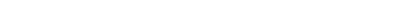The reluctance of a straight cut core is: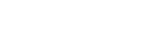The reluctance of a Cut Angle core is: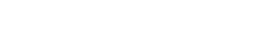The current acquired by air gap of a straight cut core is: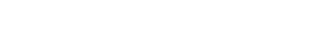The current acquired by air gap of a Cut Angle core is: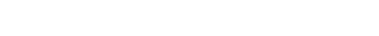Equation (3) above shows the current acquired by air gap of an Angle cut core is reduced by a factor of cosa (Cosa is always smaller than 1, therefore I'gap is always smaller than I gap).

Therefore, the excitation current of a Cut Angle core becomes: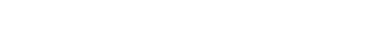We also found that cores with an angle cut dramatically reduce the acoustical noise when compared to a straight cut core, which is a very desirable property. The Cut Angle core is also applicable for Three Phase cores (Figures 5&6).The Angle-Cut core can be banded by using an "L" bracket (see Figure 7) to support the two halves from slipping during the banding process. The final assembley includes core, bobbin, "L" bracket, and band as shown in Figure 8.If you have the need for additional technical information or samples regarding this concept please contact Magnetic Metals Corporation, Anaheim, CA at (714) 828-4625.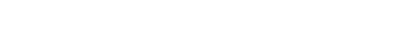The current I'gap of a core with a 45° cut is 30% less when compared to a straight cut core, and I'gap of a core with a 60° cut is less than 50% when compared to the same core having a straight cut. This we have experimentally verified.